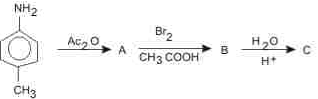Courses

# NEET UG Chemistry Mock Test 6

## 45 Questions MCQ Test NEET Mock Test Series & Past Year Papers | NEET UG Chemistry Mock Test 6

Description
This mock test of NEET UG Chemistry Mock Test 6 for NEET helps you for every NEET entrance exam. This contains 45 Multiple Choice Questions for NEET NEET UG Chemistry Mock Test 6 (mcq) to study with solutions a complete question bank. The solved questions answers in this NEET UG Chemistry Mock Test 6 quiz give you a good mix of easy questions and tough questions. NEET students definitely take this NEET UG Chemistry Mock Test 6 exercise for a better result in the exam. You can find other NEET UG Chemistry Mock Test 6 extra questions, long questions & short questions for NEET on EduRev as well by searching above.
QUESTION: 1

Solution:
QUESTION: 2

### Group reagent for the precipitation of group II basic radicals for the qualitative analysis is

Solution: KSP values of sulphides are low hence precipitated by low [S2-] ion.
HCl (with common H+ ion) decreases ionization of H2S which gives low [S2-].
Hence II group is precipitated. Others with higher KSP values not precipitated.
QUESTION: 3

### The reagent used for converting benzene to ethyl benzene is

Solution:
QUESTION: 4
100 mL of PH₃ on decomposition produced phosphorus and hydrogen. The change in volume is
Solution:
QUESTION: 5
Which of the following statements does not form a part of Bohr's model of hydrogen atom?
Solution:
QUESTION: 6
Lipids are
Solution:
QUESTION: 7
The boiling point of para nitrophenol is greater than that of ortho nitrophenol, because
Solution:
QUESTION: 8
Heat exchanged in a chemical reaction, at constant temperature and pressure, is called
Solution:
QUESTION: 9
Just before attaining equilibrium by a reversible reaction, it is found that
Solution:
QUESTION: 10
The rate of a reaction, which does not involve gases, does not depend upon
Solution:
QUESTION: 11
The internal energy of a subtance does not depend upon
Solution:
QUESTION: 12
Which of the following is the function of aspirin?
Solution:
QUESTION: 13
n-Propyl alcohol and isopropyl alcohols are examples of
Solution:
QUESTION: 14
The first ionisation potential of Li will be
Solution: As we move from left to right in a period, the ionisation potential increases with increasing atomic number and the ionisation potential keeps on decreasing regularly as we move down a group. Thus the first ionisation potential of Li is less than Be and higher than Na.
QUESTION: 15

When SCN- is added to an aqueous solution of Fe(NO3)3, the complex ion produced is

Solution:
QUESTION: 16
Lanthanides and actinides resemble in
Solution:
QUESTION: 17
A smuggler could not carry gold by depositing iron on the gold surface, because
Solution:
QUESTION: 18
Dalton's law of partial pressure is not applicable to
Solution:
QUESTION: 19
The underlining of blast furnace made of
Solution:
QUESTION: 20
Which of the following compounds has the lowest anion to cation size ratio?
Solution:
QUESTION: 21
Which of the following compounds dissolve in both HCl and NaOH solutions
Solution:
QUESTION: 22
A pseudo solid is
Solution:
QUESTION: 23
Which of the following fluorides does not exist ?
Solution:
QUESTION: 24
Hypo is used in photography to
Solution:
QUESTION: 25
One mole of fluorine is reacted with two moles of hot and concentrated KOH. The products formed are KF, H₂O and O₂. The molar ratio of KF, H₂O and O₂ respectively is
Solution:
QUESTION: 26
Which of the following is formed when Mg reacts with dilute HCl?
Solution:
QUESTION: 27

Which of the following species participate in sulphonation of benzene ring?

Solution:
QUESTION: 28
Calculate the normality of 10 volume H₂O₂?
Solution:
QUESTION: 29
A solution of NH₄Cl is
Solution:
QUESTION: 30
How many carbon atoms in the molecule HOOC-(CHOH)₂-COOH are asymmetric?
Solution:
QUESTION: 31
An aromatic amine(A) was treated with alcoholic potash and another compound(Y) when foul smelling gas was formed with formula C₆H₅NC. Y was formed by reacting a compound(Z) with Cl₂ in the presence of slaked lime. The compound(Z) is
Solution:
QUESTION: 32The final produce 'C' in the above reaction is

Solution:
QUESTION: 33
Weakest acid among the following is
Solution:
QUESTION: 34
The addition of HBr to alkenes in the presence of peroxides is called :
Solution:
QUESTION: 35
Which of the following is odd one out?
Solution:
QUESTION: 36
The most electropositive amongst the alkaline earth metals is
Solution: The electronegativity values for the elements decrease down a group. This is because as we move down the group atomic radius as well as screening effect increases. Thus, barium (Ba) is the least electronegative or most electropositive among the given alkaline earth metals.
QUESTION: 37
Which of the following is an example of synthetic polymer?
Solution:
QUESTION: 38
If a sample of an organic substance is heated strongly with soda-lime in a test tube, then ammonia is evolved. It indicates the presence of
Solution:
QUESTION: 39
In which of the following compounds transition metal is in oxidation state zero ?
Solution:
QUESTION: 40
The presence of F-centres in the crystal makes it
Solution:
QUESTION: 41
The number of gram equivalents present in 60 ml of 4 N solution is
Solution:
QUESTION: 42
Optical isomerism is shown by:
Solution:
QUESTION: 43

Liguid benzene burns in oxygen according to 2C6H6(l) + 15O2(g)→ 12CO2(g) + 6H2O(g) . How many litres of oxygen are required for complete combustion of 39 gm of liquid C6H6 (At. wt.of C=12,O=16)

Solution:
QUESTION: 44
In chemisorption, adsorption decreases after increase due to
Solution:
QUESTION: 45
The correct formula of permanganic acid is
Solution: